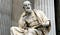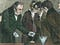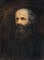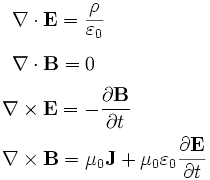# THE MAGNETIC CHARM OF WAVES

This article is aimed primarily at non-physicists wishing to have a better understanding of one of the great revolutions in science, which set the stage for modern physics. Advanced mathematics is avoided for the most part except in Maxwell, where the connection of mathematical formalism and physical reality leads to a deeper understanding of both.

In 1842 an Austrian physicist named Johann Christian Doppler proposed a theory that the frequency of a wave changes when the source of the wave is moving relative to an observer. The frequency is higher during the approach of the source to the observer and lower during the recession.

Think for a moment that you are standing on a sidewalk as an ambulance is approaching at high speed. The sound from the ambulance’s siren is a high pitched sound but gets even higher as the ambulance gets closer. The sound reaches a screeching pitch just when the ambulance passes in front of you and then the pitch begins to get lower as the ambulance is moving away. This is the Doppler effect and we have all experienced it but probably did not pay much attention.

Assume that the ambulance is stationary at a distance of 340 metres from you and its siren is turned on. The first sound wave will reach you exactly one second after its emission from the siren, because we know that sound travels at 340 metres per second. The second sound wave will hit you exactly one second later, and so on. You will hear a new sound wave per second. Now, if the ambulance begins to move toward you, the first sound wave at time zero will reach you in one second. But the second sound wave will reach you in something less than a second, as it has to travel a shorter distance now that the ambulance is closer. The frequency that you hear is higher than the frequency of emission. As the ambulance approaches, each sound wave is reaching you at much shorter intervals and each second now has several successive sounds. The siren produces sound waves at a fixed frequency but the frequency that you hear is much higher as the siren approaches. The Doppler effect applies to all waves, including light waves emitted by a source that is moving relative to an observer. Astronomers actually use the Doppler effect to determine the velocity of stars by analyzing their frequency spectra.

For its impact on our daily lives, electricity is the greatest discovery of all time. Our society has been so transformed and become so dependent on electricity, that the very survival of our large urban populations would be at stake if electricity were to disappear all of a sudden. Aside from its practical applications, the discovery of electricity has led to an extraordinary expansion of our knowledge of the universe.

The first recorded scientific observations of electricity date back to the sixth century BC, when Thales of Miletus, a Greek philosopher, experimented on static electricity produced by rubbing amber, a fossilized tree resin. In fact, the word “electricity” comes from “elektron”, the Greek word for “amber”. But it was not until the eighteenth century that we saw the first scientific breakthroughs. American scientist and scholar Benjamin Franklin conducted in 1752 his famous experiment with the kite and proved that lightning is a huge electrical spark.Thales of Miletus

Magnetism had been investigated in the sixteenth century by the English natural philosopher William Gilbert but it would take another three centuries before a complete theory would be developed to describe magnetism, electricity and the relation between them. The man who accomplished this was James Clerk Maxwell, a Scottish mathematician and theoretical physicist. Maxwell is one of the greatest scientists who ever lived. Einstein has said that “Maxwell’s work is the most profound and the most fruitful that physics has experienced since the time of Newton”.

Electricity and magnetism were thought to be two entirely different phenomena until one evening in April 1820 when a remarkable discovery showed a strong interaction between these two fascinating forces. A Danish physicist named Hans Christian Oersted was preparing to give a lecture at the University of Copenhagen. While organizing his lecture materials, Oersted noticed that a magnetic compass was deflected when he switched an electric current from a battery. In addition to being a physicist, Oersted was also a Kantian philosopher and his mind was fertile ground for processing ideas about the unity of natural phenomena.Oersted demonstrates his discovery

Oersted’s intuition made him realize that an electric current radiates a magnetic field but he needed more than intuition, he needed scientific proof. He embarked on a series of experiments and investigations and published his findings, proving that an electric current produces a magnetic field as it flows through a wire. In honour of his contributions, the oersted is the unit by which we measure magnetic induction today. Oersted’s discovery had the makings of a true scientific revolution as it created a great deal of excitement and set the stage for more intensive research in the scientific community. Oersted did not provide a mathematical formulation of the relationship between an electric current and the magnetic field it creates but a French physicist and mathematician named Andre-Marie Ampere was up to the task.

Ampere was a polymath well versed in history, poetry, philosophy and the natural sciences. He became professor of philosophy and astronomy at the University of Paris and began experimenting with electricity and magnetism when he heard of Oersted’s discovery. Ampere expanded Oersted’s experimental work and was able to prove that two parallel wires carrying electric currents attract or repel each other, depending on whether the currents flow in the same or opposite directions respectively. Ampere was not just a skillful experimenter but also an excellent physicist and mathematician who could generalize the phenomenon into a law of physics and determine the mathematical relations. Ampere’s Circuital Law relates the magnitude and direction of a magnetic field to the magnitude and direction of the electric field that produces it. The law is a cornerstone of electrodynamics and led to Maxwell’s complete formulation of electromagnetic theory.

The world of physics was now well on its way to a unification of the two great forces of electricity and magnetism. An English scientist named Michael Faraday conducted a series of experiments that lasted an entire decade, from 1821 to 1831, and discovered that a changing magnetic field induces an electric current in a circuit that encloses the magnetic field. This is known as electromagnetic induction and it is the discovery that led to the development of electromagnetic devices, such as transformers, generators and motors.

Ampere’s and Faraday’s discoveries showed that two different phenomena of nature are actually one and the same phenomenon with two distinct manifestations. Electricity and magnetism exist together, as one causes the other. This was a major scientific breakthrough that led not only to Maxwell but also to relativity and quantum physics. The discovery of electromagnetism is one of the great scientific revolutions of all time.

James Clerk Maxwell was born to a prosperous family in Edinburgh in 1831. He lost his mother to cancer when he was eight years old and his father took responsibility for the boy’s education. Maxwell was fascinated with mathematics and geometry and wrote his first mathematical paper when he was 14. He enrolled in the University of Edinburgh where he studied logic and metaphysics, mathematics and natural philosophy. Upon the completion of his studies at Edinburgh he enrolled at the University of Cambridge. At this time he already had a reputation as a talented mathematician. Maxwell presented a paper to the Royal Society titled Experiments on Colour and before long he was appointed professor of natural philosophy at Marischal College in Aberdeen and eventually appointed to a professorship at King’s College in London. While at King’s College he investigated issues in optics, astronomy, electricity and magnetism. He published a textbook titled Treatise on Electricity and Magnetism in 1873 where he distilled all that was known about electricity and magnetism in a set of four equations.James Clerk Maxwell

This set of equations is a true intellectual masterpiece and is probably the most compact and elegant set of equations in all physics. We are going to take a look at the equations, one by one, and will see that they are not as difficult to understand as they appear on a first viewing. We will also make an attempt to understand what the physical meaning of each equation is. The laws of nature can be described in ordinary language or in mathematical language. There are pros and cons in each of these methods. Both can give us insights into reality.

A mathematical equation is tautological by definition. The left hand side is the same as the right hand side. That is the very definition of a tautology. That means that a mathematical expression without empirical input reveals nothing about nature. In the best scenario, we will have an equation that tells us about quantitative relationships and helps our thinking to compare and discover new relationships between abstract forms. Thankfully, Maxwell’s equations are endowed with plenty of empirical input and his mathematical formalism has a lot to do about nature.Maxwell’s four equations

On the right hand side of the first equation we see the Greek letter ρ, which stands for the electric charge density at a point in space. The denominator stands for the permittivity of free space, which is a constant number and is equal to 8.854 x farads per meter. The permittivity of a material depends on how the material responds to an electric field. Non-conducting materials have much smaller permittivities than conducting materials. On the left hand side of the equation we see the electric field E, shown in bold to indicate a vector. A complete description of a vector always needs two numbers, magnitude and direction. (For example, velocity is a vector.) The inverted triangle with the dot to the left of the electric field E is the divergence operator. It is a mathematical operator, like the multiplication sign, just a bit more interesting. The divergence measures the extent to which the vector E behaves as a source or a sink at a given point. If the divergence is nonzero at some point, then there is a source or a sink at that point. The equation tells us that the only places at which the divergence of the electric field is not zero are those locations at which charge is present. If positive charge is present, the divergence is positive, meaning that the electric field tends to flow away from that location. If negative charge is present, the divergence is negative, and the field lines tend to flow toward that point. The first equation is also known as Gauss’s law. Basically it tells you that the presence of an electric charge gives rise to an electric field. The law is named after Carl Friedrich Gauss who formulated it in 1835 but did not publish it until 1867.

The second equation is even more compact than the first. Here we have the same divergence operator as before but here it is acting on vector B, which stands for magnetic field. This equation tells us that the divergence of the magnetic field is zero everywhere. The first equation told us that the divergence of the electric field at any point is zero unless there is an electric charge at that point. The second equation is telling us that the divergence of a magnetic field at any point is zero no matter what. We can understand this equation if we remember that all magnets are made up of two poles, north and south. There is no such thing as an isolated magnetic pole. The magnetic field flux lines depart from the north pole and end in the south pole. No concentration of “magnetic charge” is allowed at any point and none has been found in nature. The amount of incoming field at any point is exactly the same as the amount of outgoing field. The second equation is also known as Gauss’s law for magnetism. We can express the first two equations in a slightly different and less rigorous way: the basic entity for electricity is the electric charge and the basic entity for magnetism is the magnetic dipole.

The third equation tells us that electricity and magnetism always co-exist. On the left hand side we have the electric field vector E with our mathematical operator which is no longer the divergence but the curl operator . As we might suspect from its name, the curl of a vector is a measure of the field’s tendency to circulate around a point. It is almost the opposite of the divergence, which is a measure of the field’s tendency to flow away from the point. The right hand side represents the rate of change of the magnetic field B over time. The meaning of the whole equation is that a circulating electric field is produced by a magnetic field that changes over time. Wherever a changing magnetic field exists, a circulating electric field is induced. Unlike fields that originate from electric charges, induced fields have no origination or termination points, they circulate back on themselves. This equation is also known as Faraday’s law of induction. This law was discovered independently by Michael Faraday in 1831 and Joseph Henry in 1832.

Faraday referred to lines of force when he tried to explain electromagnetic induction. Scientists of his day widely rejected his ideas because they were not formulated mathematically, until Maxwell came along and developed his electromagnetic theory based on ideas from Faraday, Gauss and others. Faraday’s law of induction is captured extremely well in this compact and elegant third Maxwell equation. This ability of changing magnetic fields to induce electric fields is what makes possible the operation of transformers, electric motors and generators.

In the fourth equation we have on the left side the curl of the magnetic field B and on the right side we find what causes this curl, what causes our magnetic field at a point to start rotating about that point. As we see on the right side, it is the sum of the current density J and the term , which indicates how the electric field E changes with time. There is a new constant in addition to our familiar constant from the first equation , the permittivity of free space. The new constant is the Greek letter μ, which is the permeability of free space. The magnetic permeability indicates a material’s response to an applied magnetic field. In this case we have vacuum as our material and that’s why our permeability constant has the subscript zero.

The meaning of the fourth equation is that a circulating magnetic field is caused by the presence of an electric current and/or an electric field that changes with time. This equation is also known as the Ampere-Maxwell law, named after Andre-Marie Ampere, who had determined in 1820 the relationship between electric currents and magnetic fields. By the time Maxwell began his work in the 1850’s Ampere’s law was already well known but its applicability was limited to static situations involving steady currents. Maxwell extended the applicability to time-varying conditions by adding another source, the term in the equation.

In this discussion of Maxwell’s equations we have used the term “field” without defining it. Everyone is familiar with the term but the concept is not as clear as it seems, even to physicists. For our purposes we will define it as a region in space and time where a force exists, caused by a mass, an electric charge, a source of magnetism or a nuclear source. This definition covers all known fields in physics. If any new types of fields are discovered, the definition will need to change. One of the difficulties with the field concept is that a force is created from a source and acts at some distance without any connection to the source and without an apparent need for a transmitting medium. This is still an unresolved mystery in physics.

Maxwell’s four equations describe electric and magnetic fields in a most compact and elegant formalism but they tell us nothing about the effects of fields on electric charges. This gap is filled by the Lorentz Force equation. It is not possible to derive the Lorentz Force Law from Maxwell’s equations, unless certain strong postulates are stated. We may therefore include it with Maxwell’s equations and then the five equations will provide a complete statement of the classical theory of electromagnetism. The Lorentz Force Law describes the force experienced by an electric charge in the vicinity of an electromagnetic field. The force on the charge is given by F = q (E + v x B), where q is the electric charge, while E, v, B are the electric field, velocity and magnetic field vectors respectively. We see that the force has two components. One arises from the electric field, the other from the magnetic field.

We have written the four Maxwell equations in their differential form and completed the picture of electromagnetism with the Lorentz Force equation. Those who are mathematically inclined may want to take a look at the integral form of Maxwell’s equations as well. Their meaning is exactly the same no matter which form we choose.

It has been well worth our effort to get into the mathematics, which is not very difficult after all. The mathematical formalism becomes easier to understand once we relate it with physical meanings and describe it in plain language. In all their simplicity, compactness and elegance, Maxwell’s equations have linked electricity and magnetism with geometry, topology and physics and have redefined our perception of space and nature.

Independent consultant and author who writes about the philosophy of science and the scientific method. His most recent book is “The Mind of Science”.

## More from Michael Sidiropoulos

Independent consultant and author who writes about the philosophy of science and the scientific method. His most recent book is “The Mind of Science”.